Match Fishtank is now Fishtank Learning!

# Geometry

## Objective

Use equations to solve for unknown angles. (Part 1)

## Common Core Standards

### Core Standards

?

• 7.G.B.5 — Use facts about supplementary, complementary, vertical, and adjacent angles in a multi-step problem to write and solve simple equations for an unknown angle in a figure.

?

• 4.MD.C.5

• 4.MD.C.7

## Criteria for Success

?

1. Identify angle relationships in angle diagrams involving vertical, supplementary, and complementary angles.
2. Write equations to represent relationships between known and unknown angle measurements.
3. Determine the measures of unknown angles and judge the reasonableness of the measures.

## Tips for Teachers

?

• Lessons 3 and 4 present students with more complicated line and angle diagrams where they use various relationships between the angles to set up equations and solve for unknown angle measurements. The Guiding Questions ask students to observe and describe angle relationships they see in the diagrams first. This supports students in making sense of the diagrams and seeking a possible entry point before jumping in (MP.1).
• Some students may struggle with identifying the relevant relationships and setting up a corresponding equation; others may struggle with solving the equations. Keep an eye out for both/either struggles in order to address incorrect answers appropriately.

#### Remote Learning Guidance

If you need to adapt or shorten this lesson for remote learning, we suggest prioritizing Anchor Problem 1 (benefits from worked example). Find more guidance on adapting our math curriculum for remote learning here.

#### Fishtank Plus

• Problem Set
• Student Handout Editor
• Vocabulary Package

## Anchor Problems

?

### Problem 1

Three lines intersect at a point, as shown in the diagram below.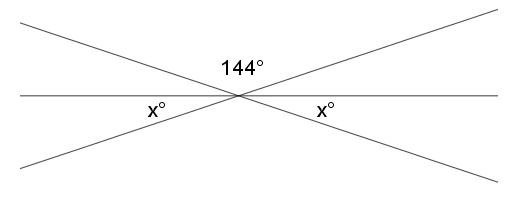Write and solve an equation to find the value of $x$

### Problem 2

Angle ${ABE}$ below measures 146°.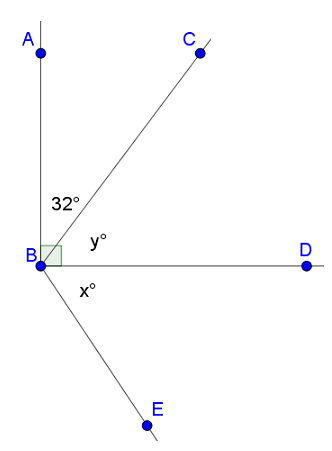Write and solve equations to determine the values of $x$ and $y$.

### Problem 3

Two lines meet at a point that is also the vertex of an angle.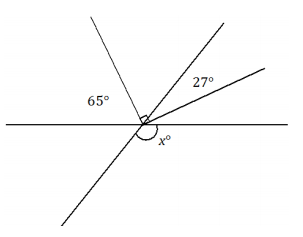Set up and solve an equation to find the value of $x$. Explain why your answer is reasonable.

#### References

EngageNY Mathematics Grade 7 Mathematics > Module 6 > Topic A > Lesson 2Exit Ticket

Grade 7 Mathematics > Module 6 > Topic A > Lesson 2 of the New York State Common Core Mathematics Curriculum from EngageNY and Great Minds. © 2015 Great Minds. Licensed by EngageNY of the New York State Education Department under the CC BY-NC-SA 3.0 US license. Accessed Dec. 2, 2016, 5:15 p.m..

### Problem 4

Two lines meet at a point that is also a vertex of an angle; the measurement of ${\angle AOF}$ is 134°.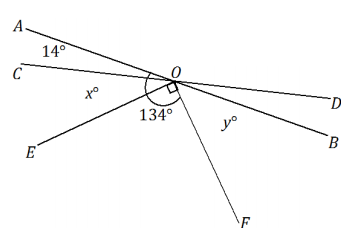Set up and solve an equation to find the values of $x$ and $y$.

#### References

EngageNY Mathematics Grade 7 Mathematics > Module 6 > Topic A > Lesson 3Opening Exercise

Grade 7 Mathematics > Module 6 > Topic A > Lesson 3 of the New York State Common Core Mathematics Curriculum from EngageNY and Great Minds. © 2015 Great Minds. Licensed by EngageNY of the New York State Education Department under the CC BY-NC-SA 3.0 US license. Accessed Dec. 2, 2016, 5:15 p.m..

## Problem Set

?The following resources include problems and activities aligned to the objective of the lesson that can be used to create your own problem set.

?

Two lines intersect at a point that is also a vertex of an angle.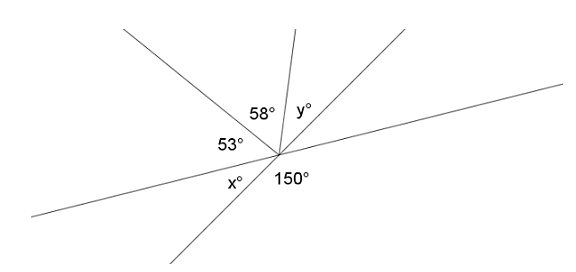Write and solve equations to determine the values of $x$ and $y$.

?